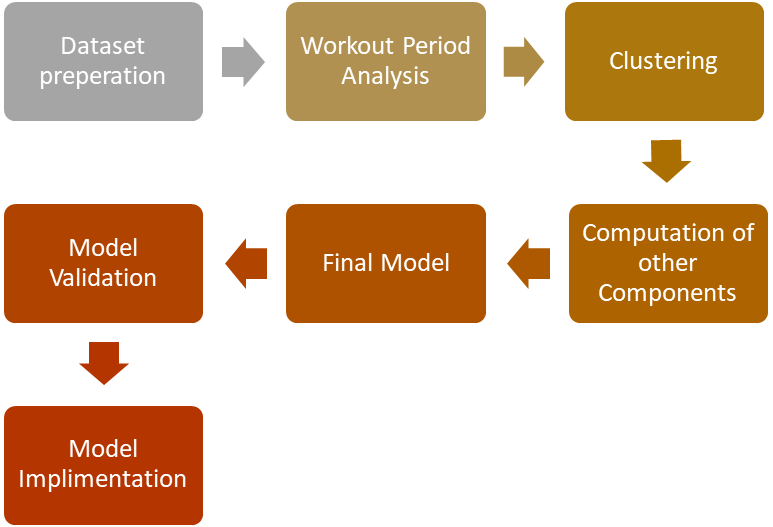## Loss Given Default (LGD) Modelling for Banks

### Background

Problem Statement
To identify the estimated potential credit losses, we calculate a loan’s projected profitability. Under IFRS 9, banks are required to recognize credit losses at all times based on reasonable information, considering past, current, and future events. Typically, most, if not all, models for expected credit loss (ECL) are developed using the past information and performance of existing and former clients. Given this assumption, banks need to update their models regularly to be able to accurately capture the more recent and current performance of their portfolio.

A. Loss Given Default (LGD)

Loss Given Default (LGD) is a lender’s (or creditor’s) projected loss if a borrower triggers an event of default. LGD is a measure used by Financial Institutions and other private, non-bank lenders to help calculate the projected loss of a loan (often referred to as a “credit facility,” which may include operating credit, term loans, commercial mortgages, capital leases, etc.). LGD is most commonly expressed as a percentage; however, it can also be expressed as an absolute dollar figure. It is tied very closely to any security underpinning credit exposure. In fact, in its most literal sense, LGD is the inverse of an asset’s recovery rate.

B. Calculations of LGD

LGD is calculated as 1 minus the anticipated recovery rate of an asset (or assets). The recovery rate (expressed as a percentage) is the proportion of an asset’s value that a lender can realistically expect to get back if a client were to trigger an event of default that eventually leads the creditor to a liquidation scenario. If the expected recovery rate of an asset (or asset class) is 78% (0.78), the LGD is (1 – 0.78), or 22%. A higher anticipated recovery rate equals a lower LGD.

LGD= 1- Recovery Rate

IFRS 9 Expected Credit Loss is calculated as follows:

PD stands for “Probability of Default.”

LGD stands for “Loss Given Default.”

EAD stands for “Exposure at Default.”

Discounting is for time value of money

Approach & Output:1. Dataset Preparation

Model development starts with dataset preparation, which includes identifying data sources, resolving data issues, cleaning datasets, generating the base dataset, i.e., the first default dataset, and applying exclusions, i.e., accounts with a default balance of 0 were not included.

2. Workout Period Analysis:

The second step involves performing workout period analysis. Workout period has to be identified based on the time taken to cure most of the loans recoverable. A period of three years is usually good.

3. Clustering

In clustering, datasets are divided into different groups based on their similarities. This aids in generalizing certain behaviours across a specific grouping. Mostly, we use the clustering technique for the analysis. i.e., K-Means clustering – one of the simplest unsupervised machine learning algorithms. In a nutshell, K-means clustering identifies k numbers of centroids (means) and allocates every data point to the nearest cluster while minimizing the distances of the data points from the centroids. LGD can be obtained for different clusters/segments of customers.

4. Interest Rate for Recovery Discounting

In Time Value of Money (TVM), discounting is defined as the process of converting a value received in a future time to an equivalent value received immediately.

5. Direct Cost

Direct cost percentage is derived by dividing the direct cost as of a particular report month by the outstanding balance of the portfolio as of that particular report month.

6. Non- Cure Probability

The approach to cure probability is if the default facilities cure their status and return to non-default status, then LGD becomes zero. Based on the samples, the facilities were defined as cured if they return to non-default status and keep the non-default status for 6 months or longer. The total number of non-cured accounts divided by the total number of accounts in the sample is non-cure probability.

7. Output

Finally, the loss given default is obtained by combining: 1. Recovery during workout period 2. Non-Cure probability 3. Discounting the recovery (TVM). LGD can be obtained for various customer segments. Segments can be obtained by data-based clustering or business logic. The LGD thus obtained can be projected into the future using macro-economic adjustments. This will eventually be used in ECL calculations and loss mitigation strategies.

Follow